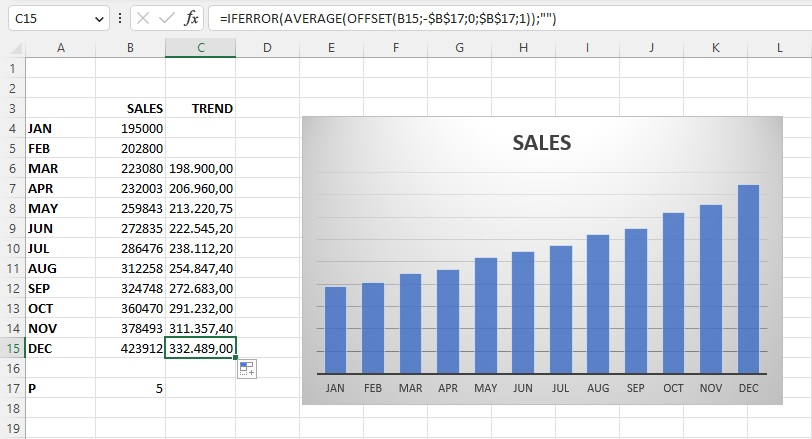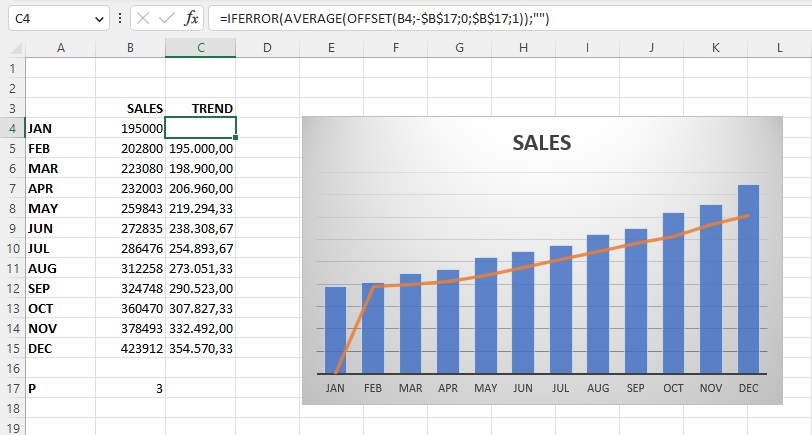# Moving Average

The moving average is a statistical method by which we track the average change in data over time. We can use it to calculate the trend of data change. Although it already exists within the trendline and can be applied automatically, in the following text you will learn how to calculate it manually, and you will better understand this method and know when and how to apply it.

We will start from a simple table in which sales can be seen during 12 months of a year. Based on this table, we created a Column Chart.We will add another column and name it “TREND” and this is where the moving average will be calculated. It can be presented as simple and exponential. In this text, we will describe how to calculate a simple moving average, which is the sum of average values divided by the number of periods or the average of values for a given number of periods. We will enter the formula in cell C4:

=AVERAGE(OFFSET(B4,-\$B\$17,0,\$B\$17,1))

This formula needs to be “framed” using the IFERROR function, which gives us the final version of the formula:

=IFERROR(AVERAGE(OFFSET(B4,-\$B\$17,0,\$B\$17,1)),””)If you pay attention, you will see that the formula refers to the number of periods, contained in cell B17. We fixed this address so that it does not change when copying the formula. The initial value was 5, we will change it to 3.

Next, we will add the trend column as an additional series to the chart. We will convert it into a Combo Chart, and we will show the trend with an orange line.If we change the value of cell B17, the number of periods will also change. In essence, the more periods the trend line has, the more accurate it will be, but it will only be displayed correctly after a given number of periods. Feel free to test the model!